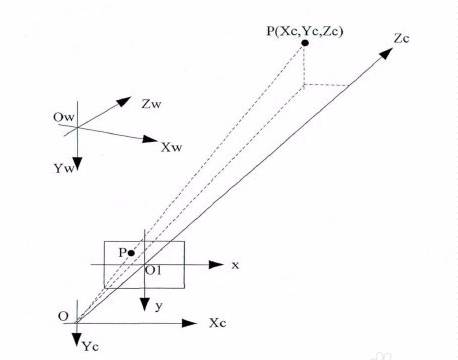$$\frac{x}{X}=\frac{y}{Y}=\frac{f}{Z}(1)$$

$$\frac{u_x-x_0}{X}=\frac{y_0-u_y}{Y}=\frac{f}{Z}(2)$$

$$u_x = X\frac{f}{Z}+x_0->Zu_x=Xf+Zx_0(3)$$
$$u_y = -Y\frac{f}{Z}+y_0->Zu_y=-Yf+Zy_0(4)$$

$$Z(u_x,u_y,1)= \begin{bmatrix}1&0&0\\ 0&-1&0\\ 0&0&1\end{bmatrix}\cdot \begin{bmatrix}f&0&x_0\\ 0&f&y_0\\ 0&0&1\end{bmatrix}\cdot \begin{bmatrix}X\\ Y\\ Z\end{bmatrix}(5)$$

$$\begin{bmatrix}X_w\\ Y_w\\ Z_w\end{bmatrix}= \begin{bmatrix}a_1&a_2&a_3\\ b_1&b_2&b_3\\ c_1&c_2&c_3\end{bmatrix}\cdot \begin{bmatrix}X\\ Y\\ Z\end{bmatrix}+ \begin{bmatrix}X_s\\ Y_s\\ Z_s\end{bmatrix}(6)$$